# Avg speed of flight

The students vice adventure had a 2,367 km flight. If they travel time was 2 hours and 56 minutes, what was their average speed in kilometres per hour?

Result

v =  806.932 km/h

#### Solution:Leave us a comment of example and its solution (i.e. if it is still somewhat unclear...):

Showing 0 comments:Be the first to comment!## Next similar examples:

1. Speed of carIn 2 hours 40 mins, a car travels 100km. At what speed is the car traveling?
2. Speeds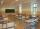George pass on the way to school distance 200 meters in 165 seconds. What is the average walking speed in m/s and km/h?
3. Two cars 2Two cars started from two positions 87 km distant at the same time in opposite directions at speeds 81 km/h and 75 km/h. What was the distance between them after 2 hours 50 minutes of driving.
4. HoursThe lesson lasts 45 minutes. For the week, students have 18 lesson hours. How many are the actual hours?
5. Bean bag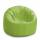A student tossed a bean bag. It landed 216 inches away. How many yards are equal to 216 inches?
6. Bus 14Boatesville is 65.35 kilometers from Stanton. A bus traveling from Stanton is 24.13 kilometers from Boatesville. How far has the bus traveled?
7. Hr to minSue biked to school in 5/12 of an hour. How many minutes did it take her to ride to school?
8. The resultHow many times I decrease the number 1632 to get the result 24?
9. Addition of Roman numbersAdded together and write as decimal number: LXVII + MLXIV
10. CakesOn the bowl were a few cakes. Jane ate one-third of them, Dana ate a quarter of those cakes that remained. a) What part (of the original number of cakes) Dana ate? b) At least how many cakes could be (initially) on thebowl?
11. If-then equationIf 5x - 17 = -x + 7, then x =
12. Ten pupils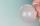10 pupils went to the store. 6 pupils bought lollipops and 9 pupils bought chewing gum. How many pupils have bought both lollipops and chewing gums (if everyone bought something)?
13. Plane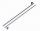On how many parts divide plane 6 parallels?
14. Expressions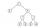Find out value of expressions if a = -1, b =2: x=b - 2a - ab y=a3 - b2 - 2ab z=a2 b3 - a3 b2 w=a + b + a3 - b2
15. Line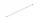How many parts of line divide 5 (different) points that lie on it?
16. Flood waterFlood waters in some US village meant that the homes had to evacuate 364 people. 50 of them stayed at elementary schools, 59 them slept with their friends and others went to relatives. How many people have gone to relatives?
17. AreaCalculate: ?